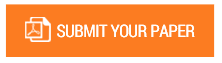Determination of Combination of Input Values of Simulated Motor Using Taguchi Method

Authors : Ariunbolor Purvee; Purevdorj Batkhuu

Volume/Issue : Volume 6 - 2021, Issue 4 - April

Scribd : https://bit.ly/34Pi73v

The most popular type of motors in use today is squirrel-cage induction motors. All types of motor faults can be studied if a motor could be simulated based on the motor without faults. The goal of this study was to determine the input optimum values for making it almost identical values of outputs f the simulated motor to the target values on the nameplate data of the actual motor. In this study, the Taguchi method, calculated using MATLAB, was used to optimize the values input of the simulated motors. For dynamic simulation purposes, inputs and outputs of a simulated motor need to be selected. In this study, stator resistance, stator inductance, rotor bar resistance, rotor bar inductance, rotor end ring resistance, and rotor end ring were selected as the input parameters. Rotations per minute (rpm), torque, and stator current were selected as output parameters; they are also provided on the nameplate data on the actual motor. These results allow us to develop dynamic simulations to study a variety of motor faults and to compare the results of a simulated motor with and without faults, to better understand and analyze the frequency domain, determine the root cause of the vibrations. All code work is shown in the paper and one new formula was developed based on this study.

Keywords : Model, Simulation, Optimization, Modelling, Dynamic Simulation, MATLAB.

#### CALL FOR PAPERS

Paper Submission Last Date
31 - May - 2022